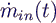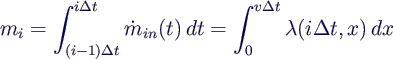### Modeling of a single conveyor

• Simple discrete-event model:
•• standard component in commercial simulation environments
• Transportation of dry bulk material:
• incoming mass flow→ line load•• for constant v
•• Basic modeling approach (for constant v):
• conveyor divided into compartments of equal length lE = v ∆t
• discrete entities Ei with mass mi
•• for simplicity: choose lE = l/N
•• alternatively: compartments of equal mass
• more complex timing of events
• hard to implement in some environments (e. g. Arena)